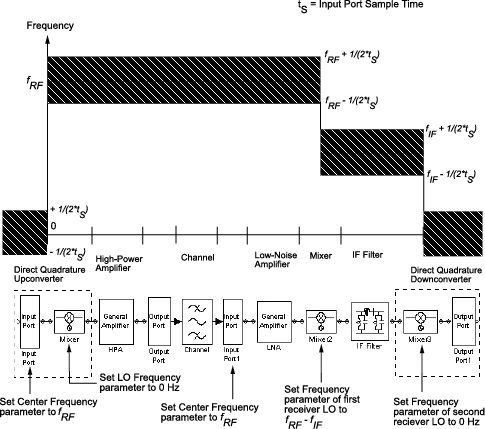## Model a Mixer Chain

RF Blockset™ Equivalent Baseband software uses a baseband equivalent model to simulate RF components in the time domain. The blockset only models a band of frequencies around the carrier frequency of each component; the frequency band is determined by the following parameters of the corresponding Input Port block:

• Reciprocal of the Sample time (s)

• Center frequency (Hz)

When a mixer is present in a physical subsystem, it shifts the carrier frequency of the signal. This shift affects the frequencies that are used to create baseband equivalent model.

To illustrate how the mixer works, consider a typical RF mixer chain that consists of the following components:

• High-Power Amplifier

• Channel

• Low-Noise Amplifier

• Downconverting Mixer

• IF Filter